Courses

# Test: Redox Reactions - From Past 28 Years Questions

## 16 Questions MCQ Test Chemistry Class 11 | Test: Redox Reactions - From Past 28 Years Questions

Description
This mock test of Test: Redox Reactions - From Past 28 Years Questions for NEET helps you for every NEET entrance exam. This contains 16 Multiple Choice Questions for NEET Test: Redox Reactions - From Past 28 Years Questions (mcq) to study with solutions a complete question bank. The solved questions answers in this Test: Redox Reactions - From Past 28 Years Questions quiz give you a good mix of easy questions and tough questions. NEET students definitely take this Test: Redox Reactions - From Past 28 Years Questions exercise for a better result in the exam. You can find other Test: Redox Reactions - From Past 28 Years Questions extra questions, long questions & short questions for NEET on EduRev as well by searching above.
QUESTION: 1

### Which substance serves as reducing agent in the following reaction ?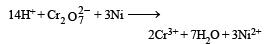Solution:

The element undergo oxidation itself and reduces others is known as reducing agent.
In this reaction O. N. of Ni Changes from 0 to + 2 and hence Ni acts as a reducing agent.

QUESTION: 2

### Phosphorus has the oxidation state of + 3 in

Solution:

O.N. of P in H3PO3 (phosphorous acid) 3 × 1 + x + 3 × (– 2) = 0 or x = + 3 In orthophosphoric acid (H3PO4) O.N. of P is + 5, in hypophosphorous acid (H3PO2) it is + 1 while in metaphosphoric acid (HPO3), it is + 5,

QUESTION: 3

### In which of the following reactions, there is no change in valency ? 

Solution: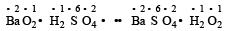In this reaction, none of the elements undergoes a change in oxidation number or valency.

QUESTION: 4

The oxidation number of chromium in potassium dichromate is 

Solution:

Let x = oxidation no. of Cr in K2Cr2O7.
∴ (2 × 1) + (2 × x) + 7 (– 2) = 0
or 2 + 2x – 14  = 0 or x = + 6.

QUESTION: 5

The loss of electron is termed as 

Solution:

Losing of electron is called oxidation,

QUESTION: 6

The oxide, which cannot act as a reducing agent, is

Solution:

Carbon has the maximum oxidation state of + 4, therefore carbon dioxide (CO2) cannot act as a reducing agent.

QUESTION: 7

Which of the following involves a redox reaction?

Solution: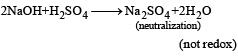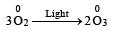(not redox reaction)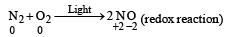here oxidation of N2 & reduction of O2 is taking place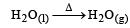(not redox reaction)

QUESTION: 8

The oxidation number of phosphorus in pyrophosphoric acid is 

Solution:

Pyrophosphoric acid H4P2O7
Let oxidation state of phosphorus is x(4 × 1 + (– 2) × 7 + 2 x) = 0
∴ 2x = 10   or  x = +5

QUESTION: 9

The following redox reaction is balanced by which set of coefficients ?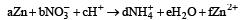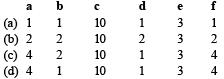Solution: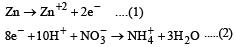operate eq. (1) × 4 + eq. (2) × 1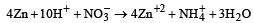QUESTION: 10

A compound contains atoms of three elements A, B and C. If the oxidation number of A is +2, B is +5, and that of C is –2, the possible formula of the compound is : 

Solution:

Oxidation number of a compound must be 0.
Using the values for A, B and C in the four options we find that A3(BC4)2 is the answer.
Check : (+2)3 + [(+5)+4(–2)]2 = 6 + (5–8)2 = 0

QUESTION: 11

Zn gives H2 gas with H2SO4 and HCl but not with HNO3 because 

Solution:

Zinc gives H2 gas with dil H2SO4/HCl but not with HNO3 because in HNO3, NO3 ion is reduced and give NH4NO3, N2O, NO and NO2 (based upon the concentration of HNO3)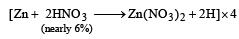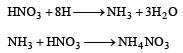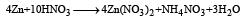Zn is on the top position of hydrogen in electrochemical series. So Zn displaces H2 from dilute H2SO4 and HCl with liberation of H2.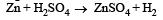QUESTION: 12

The oxidation states of sulphur in the anions SO32–, S2O42– and S2O62– follow the order 

Solution:

The correct answer is option B
Oxidation state of S2​O42−
2(x) + 4(−2) = −2
2x = 8 − 2
2x = 6
x = 3
Oxidation state of SO32−
x + 3(−2) = −2
x = 6 − 2
x = 4
Oxidation state of S2​O62−
2(x) + 6(−2) = −2
2x = 12 − 2
2x = 10
x = 5
So the oxidation state of sulphur in the anions S2​O42−​, S2​O42−​ and S2​O62−​ follows the order.S2​O42−​ < SO32−​ < S2​O62−​.

QUESTION: 13

Oxidation numbers of P in PO43 , of S in SO42– and that of Cr in Cr2O72–  are respectively 

Solution:

(i) Sum of oxidation states of all atoms = charge of ion.
(ii) oxidation number of oxygen = -2
Let the oxidation state of P in PO43- is x.
PO43-

x + 4 (-2) = - 3
x-8 = - 3
x = +5

Let the oxidation state of S in SO42- is y
y + 4(-2) = -2
y-8 = - 2
y = +6

Let the oxidation state of Cr in Cr2O72- is z.
2 x z+7(-2) = -2
2z-14 = - 2
z=+6
Hence, oxidation state of P, S and Cr are +5, +6 and +6

QUESTION: 14

When Cl2  gas reacts with hot and concentrated sodium hydroxide solution, the oxidation number of chlorine changes from : 

Solution:

The correct answer is Option B.

The reaction of chlorine gas with hot and concentrated sodium hydroxide solution is

3Cl2 ​+ 6NaOH ⟶ NaClO3 ​+ 5NaCl + 3H2​O

Oxidation number of Cl is 0 in Cl2, −1 in NaCl and +5 in NaClO3

​So, the oxidation number of chlorine changes from Zero to -1 and Zero to +5.

QUESTION: 15

A mixture of potassium chlorate, oxalic acid and sulphuric acid is heated. During the reaction which element undergoes maximum change in the oxidation number ? 

Solution: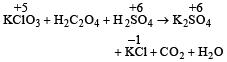i.e. maximum change in oxidation number is observed in Cl (+5 to –1).

QUESTION: 16

Standard reduction potentials of the half reactions are given below :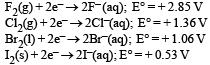The strongest oxidising and reducing agents respectively are : [2012 M]

Solution:

Higher the value of reduction potential higher will be the oxidising power whereas the lower the value of reduction potential higher will be the reducing power.